• 对称矩阵与实对称矩阵
千次阅读
2019-09-24 11:03:09

对称矩阵（Symmetric Matrices）：是指以主对角线为对称轴，各元素对应相等的矩阵。  在线性代数中，对称矩阵是一个方形矩阵，其转置矩阵和自身相等。
实对称矩阵：如果有n阶矩阵A，其矩阵的元素都为实数，且矩阵A的转置等于其本身（aij=aji）(i,j为元素的脚标），则称A为实对称矩阵。
两个概念的不同之处在于：实对称矩阵里的元素全是实数，而对称矩阵只说明A^T=A，没说明矩阵中的元素是实数，矩阵中的元素不仅可以是实数，也可以是虚数，甚至元素本身就是一个矩阵或其它更一般的数学对象，实对称矩阵就说明了矩阵中的元素要是实数。

更多相关内容
• 对称矩阵及稀疏矩阵的压缩存储 1.稀疏矩阵 　对于那些零元素数目远远多于非零元素数目，并且非零元素的分布没有规律的矩阵称为稀疏矩阵（sparse）。 　人们无法给出稀疏矩阵的确切定义，一般都只是凭个人的直觉来...
• 本文主要针对线性代数中的正定矩阵、实对称矩阵、矩阵特征值分解以及矩阵 SVD 分解进行总结。 如果你对这篇文章可感兴趣，可以点击「【访客必读 - 指引页】一文囊括主页内所有高质量博客」，查看完整博客分类与对应...

## 前言

本文主要针对线性代数中的正定矩阵、实对称矩阵、矩阵特征值分解以及矩阵 SVD 分解进行总结。

如果你对这篇文章可感兴趣，可以点击「【访客必读 - 指引页】一文囊括主页内所有高质量博客」，查看完整博客分类与对应链接。

## 正定矩阵

### 1. 概念

首先正定矩阵是定义在对称矩阵的基础上，其次对于任意非零向量 x \textbf{x} ，若 x T A x > 0 \textbf{x}^T\textbf{\textit{A}}\textbf{x}>0 恒成立，则矩阵 A \textbf{\textit{A}} 为正定矩阵；若 x T A x ≥ 0 \textbf{x}^T\textbf{\textit{A}}\textbf{x}\geq 0 恒成立，则矩阵 A \textbf{\textit{A}} 为半正定矩阵。

### 2. 物理意义

任意非零向量 x \textbf{x} 经过矩阵 A A 线性变换后，与原先向量的夹角 ≤ 90 \leq 90 度。

### 3. 其他充要条件

• 充要条件1： 矩阵 A \textbf{\textit{A}} 的全部特征值都是正数
• 推论： A \textbf{\textit{A}} 正定，则 ∣ A ∣ > 0 |\textbf{\textit{A}}|>0 ，即 A \textbf{\textit{A}} 可逆（有时会根据矩阵正定来判断是否可逆）
• 推论： A \textbf{\textit{A}} 正定，则 A \textbf{\textit{A}} 与单位阵合同，即存在可逆阵 C \textbf{\textit{C}} ，使得 C T AC = E \textbf{\textit{C}}^T\textbf{\textit{A}}\textbf{\textit{C}}=\textbf{\textit{E}} 成立
• 充要条件2： 矩阵 A \textbf{\textit{A}} 的各阶顺序主子式都是正数，即 Δ i > 0 \Delta_i>0
• 其中 Δ i \Delta_i 表示矩阵 A \textbf{\textit{A}} i i 行与前 i i 列组成的子矩阵的行列式的值
• 推论： ∣ A ∣ > 0 |A|>0 A A 一定可逆

## 实对称矩阵

### 1. 概念

矩阵为方阵，其中元素均为实数，且 A = A T \textbf{\textit{A}}=\textbf{\textit{A}}^T

### 2. 性质

• 性质1： 实对称矩阵的特征值都是实数。
• 假设 λ \lambda x \textbf{x} 分别为矩阵 A \textbf{\textit{A}} 的特征值、特征向量，即 A x = λ x \textbf{\textit{A}}\textbf{x}=\lambda \textbf{x}
• 等式两边取共轭，即 a + b i ‾ = a − b i \overline{a+bi}=a-bi A ‾ x ‾ = λ ‾ x ‾ \overline{\textbf{\textit{A}}}\overline{\textbf{x}}=\overline{\lambda} \overline{\textbf{x}} A \textbf{\textit{A}} 是实对称矩阵，因此 A = A T = A ‾ \textbf{\textit{A}}=\textbf{\textit{A}}^T=\overline{\textbf{\textit{A}}} ，即 A x ‾ = λ ‾ x ‾ \textbf{\textit{A}}\overline{\textbf{x}}=\overline{\lambda} \overline{\textbf{x}}
• 等式两边取转置，则 x T A = λ x T \textbf{x}^T\textbf{\textit{A}}=\lambda \textbf{x}^T
• x T A x ‾ = λ ‾ x T x ‾ = λ x T x ‾ \textbf{x}^T\textbf{\textit{A}}\overline{x}=\overline{\lambda}\textbf{x}^T\overline{\textbf{x}}=\lambda \textbf{x}^T\overline{\textbf{x}}
• ( λ − λ ‾ ) ∥ x ∥ 2 2 = 0 (\lambda-\overline{\lambda})\left\|\textbf{x}\right\|_2^2=0 ，由于 ∥ x ∥ 2 2 > 0 \left\|\textbf{x}\right\|_2^2>0 ，因此 λ = λ ‾ \lambda=\overline{\lambda} λ \lambda 为实数
• 性质2： 实对称矩阵不同特征值所对应的特征向量必定正交。
• 假设 A x 1 = λ 1 x 1 \textbf{\textit{A}}\textbf{x}_1=\lambda_1 \textbf{x}_1 A x 2 = λ 2 x 2 \textbf{\textit{A}}\textbf{x}_2=\lambda_2 \textbf{x}_2 成立
• x 1 T A = λ 1 x 1 T \textbf{x}_1^T\textbf{\textit{A}}=\lambda_1 \textbf{x}_1^T
• x 1 T A x 2 = λ 1 x 1 T x 2 = λ 2 x 1 T x 2 \textbf{x}_1^T\textbf{\textit{A}}\textbf{x}_2=\lambda_1 \textbf{x}_1^T\textbf{x}_2=\lambda_2\textbf{x}_1^T\textbf{x}_2
• ( λ 1 − λ 2 ) x 1 T x 2 = 0 (\lambda_1-\lambda_2)\textbf{x}_1^T\textbf{x}_2=0 ，因此 x 1 \textbf{x}_1 x 2 \textbf{x}_2 正交
• 性质3： 实对称矩阵相同特征值所对应的特征向量必定线性无关。
• 证明较繁琐，不详细展开
• 线性无关的向量可以通过施密特正交化转为正交向量
• 对于线性无关向量组 x 1 , x 2 , . . . , x n \textbf{x}_1,\textbf{x}_2,...,\textbf{x}_n ，转为正交向量组 y 1 , y 2 , . . . , y n \textbf{y}_1,\textbf{y}_2,...,\textbf{y}_n
• y 1 = x 1 \textbf{y}_1=\textbf{x}_1
• y i = x i − ∑ j = 1 i − 1 x i T y j y j T y j y j \textbf{y}_i=\textbf{x}_i-\sum\limits_{j=1}^{i-1}\displaystyle\frac{\textbf{x}_i^T\textbf{y}_j}{\textbf{y}_j^T\textbf{y}_j}\textbf{y}_j
• 由于新的正交向量都是原来线性无关向量的线性组合，而原先的线性无关向量对应的特征值均相同，因此新的正交向量也均为该相同特征值对应的特征向量
• 性质4： 任何一个实对称矩阵，都可以正交对角化。
• 正交对角化，即存在一个正交矩阵 Q ( Q T = Q − 1 ) \textbf{\textit{Q}}(\textbf{\textit{Q}}^T=\textbf{\textit{Q}}^{-1}) 使得 Q T AQ = D \textbf{\textit{Q}}^T\textbf{\textit{A}}\textbf{\textit{Q}}=\textbf{\textit{D}} ，其中 D \textbf{\textit{D}} 是一个对角矩阵
• 实对称矩阵，一定有 n n 个解，因为实对称矩阵特征值都是实数，因此一共有 n n 个实特征值（包括重特征值）—— 性质 1 1
• 不同特征值对应的特征向量正交，相同特征值也一定存在对应的正交向量 —— 性质 2 , 3 2,3
• 实对称矩阵，一定有 n n 个正交特征向量，因此可以特征值分解，即该性质成立
• 性质5： 实对称矩阵的非零特征值个数等于矩阵的秩
• 矩阵 A \textbf{\textit{A}} 相似于对角矩阵， P − 1 AP = D \textbf{\textit{P}}^{-1}\textbf{\textit{A}}\textbf{\textit{P}}=\textbf{\textit{D}}
• 对角矩阵 D \textbf{\textit{D}} 的秩 = 矩阵 A \textbf{\textit{A}} 的秩 = D \textbf{\textit{D}} 非零特征值个数
• 矩阵 A \textbf{\textit{A}} 与 矩阵 D \textbf{\textit{D}} 相似，则特征值相同
• 性质6：实对称矩阵不一定可逆，但若可逆，则一定是实对称矩阵
• 0 矩阵对称不可逆
• ( A − 1 ) T = ( A T ) − 1 = A − 1 (A^{-1})^T=(A^T)^{-1}=A^{-1}

## 矩阵特征值分解

### 1. 概念

n ∗ n n*n 的方阵 A \textbf{\textit{A}} ，由 A x = λ x \textbf{\textit{A}}\textbf{x}=\lambda \textbf{x} 可以得到 AV = V Λ \textbf{\textit{A}}\textbf{\textit{V}}=\textbf{\textit{V}}\Lambda

• 如果方阵 A \textbf{\textit{A}} n n 个线性无关的特征向量，则 V \textbf{\textit{V}} 可逆
• A = V Λ V − 1 \textbf{\textit{A}}=\textbf{\textit{V}}\Lambda\textbf{\textit{V}}^{-1}
• 其中矩阵 V \textbf{\textit{V}} 的列为方阵 A \textbf{\textit{A}} 的特征向量， Λ = d i a g ( λ 1 , λ 2 , . . . , λ n ) , λ i ≥ λ i + 1 \Lambda=diag(\lambda_1,\lambda_2,...,\lambda_n),\lambda_i\geq \lambda_{i+1}

## 矩阵 SVD 分解

### 1. 概念

任意一个矩阵 A \textbf{\textit{A}} 都可以分解为 A = U Σ V T \textbf{\textit{A}}=\textbf{\textit{U}}\Sigma\textbf{\textit{V}}^T ，其中 U , V \textbf{\textit{U}},\textbf{\textit{V}} 均为正交单位矩阵， Σ \Sigma 为对角矩阵。

### 2. 证明

• A T A = ( U Σ V T ) T U Σ V T = V Σ 2 V T \textbf{\textit{A}}^T\textbf{\textit{A}}=(\textbf{\textit{U}}\Sigma\textbf{\textit{V}}^T)^T\textbf{\textit{U}}\Sigma\textbf{\textit{V}}^T=\textbf{\textit{V}}\Sigma^2\textbf{\textit{V}}^T ，由于 A T A \textbf{\textit{A}}^T\textbf{\textit{A}} 为实对称矩阵，因此 V \textbf{\textit{V}} 为矩阵 A T A \textbf{\textit{A}}^T\textbf{\textit{A}} 对应特征向量组成的正交单位阵。
• A A T = U Σ V T ( U Σ V T ) T = U Σ 2 U T \textbf{\textit{A}}\textbf{\textit{A}}^T=\textbf{\textit{U}}\Sigma\textbf{\textit{V}}^T(\textbf{\textit{U}}\Sigma\textbf{\textit{V}}^T)^T=\textbf{\textit{U}}\Sigma^2\textbf{\textit{U}}^T ，由于 A A T \textbf{\textit{A}}\textbf{\textit{A}}^T 为实对称矩阵，因此 U \textbf{\textit{U}} 矩阵 A A T \textbf{\textit{A}}\textbf{\textit{A}}^T 对应特征向量组成的正交单位阵。
• AV = U Σ \textbf{\textit{A}}\textbf{\textit{V}}=\textbf{\textit{U}}\Sigma ，其中 Σ \Sigma 为对角阵，因此 A v i = σ i u i \textbf{\textit{A}}\textbf{v}_i=\sigma_i\textbf{u}_i ，由此可以得到对角矩阵 Σ \Sigma ，其中 σ i \sigma_i 就是奇异值。
• A m ∗ n = U m ∗ m Σ m ∗ n V n ∗ n T \textbf{\textit{A}}_{m*n}=\textbf{\textit{U}}_{m*m}\Sigma_{m*n}\textbf{\textit{V}}_{n*n}^T

### 3. 几何角度

矩阵 U , V U,V 仅负责旋转， Σ \Sigma 负责放缩，具体示意图如下：### 4. SVD 压缩

如下所示，仅选取前 r r 个不为零的奇异值，可以实现无损压缩。注意非零奇异值的个数等于矩阵 A A 的秩。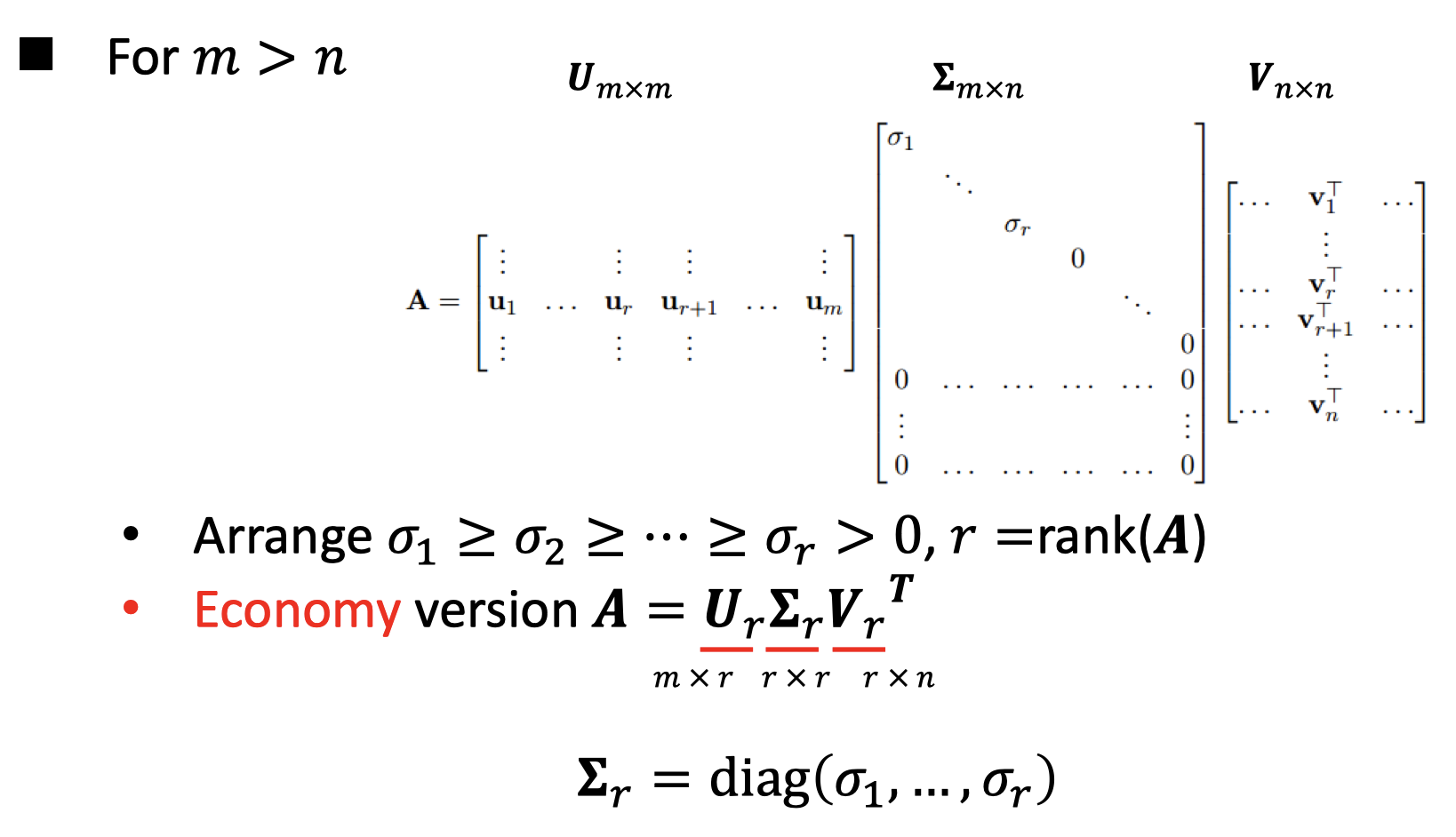### 5. 计算伪逆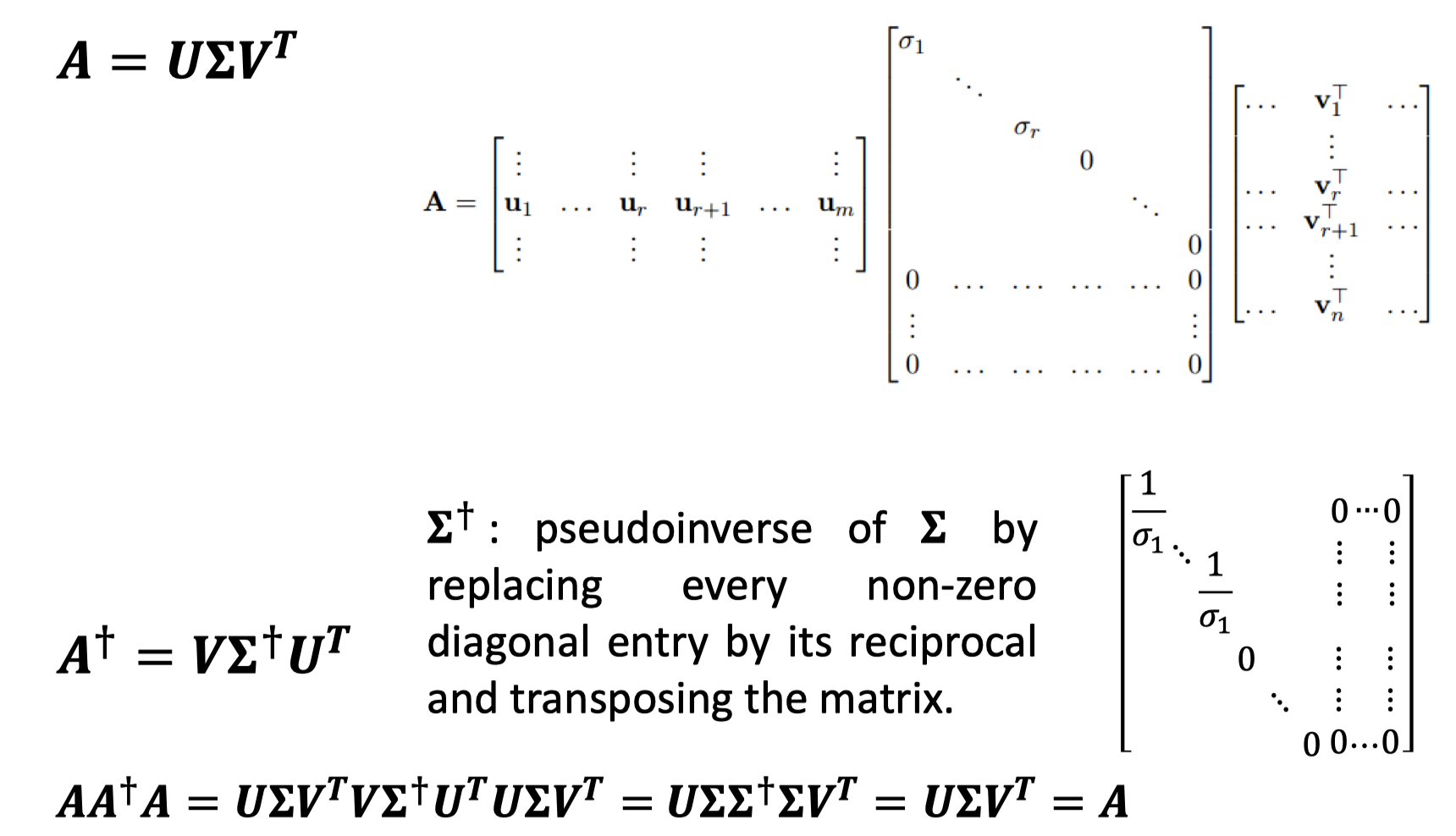### 6. Eckart-Young Theorem

如果矩阵 B \mathbf{B} 的秩为 k k ，则 ∣ ∣ A − B ∣ ∣ ≥ ∣ ∣ A − A k ∣ ∣ ||A-B||\geq||A-A_k|| 对如下三个矩阵范数成立：

• ∣ ∣ A ∣ ∣ 2 = σ 1 ||A||_2=\sigma_1 ，即最大的奇异值
• ∣ ∣ A ∣ ∣ N u c l e a r = ∑ i = 1 r σ i ||A||_{Nuclear}=\sum\limits_{i=1}^r\sigma_i
• Frobenius norm = ∣ ∣ A ∣ ∣ 2 , 1 = ∣ ∣ A ∣ ∣ F = ( t r ( A T A ) ) 1 / 2 = ( ∑ i = 1 m ∑ j = 1 n a i j 2 ) 1 / 2 =||A||_{2,1}=||A||_F=(tr(A^TA))^{1/2}=(\sum\limits_{i=1}^m\sum\limits_{j=1}^na_{ij}^2)^{1/2}

其中 A \mathbf{A} A k \mathbf{A_k} 定义如下：
A = U Σ V T = ∑ i = 1 r σ i u i v i T A k = U k Σ k V k T = ∑ i = 1 k σ i u i v i T \begin{aligned} & \mathbf{A}=\mathbf{U}\Sigma\mathbf{V}^T=\sum\limits_{i=1}^r \sigma_i\mathbf{u}_i\mathbf{v}_i^T\\ & \mathbf{A}_k=\mathbf{U}_k\Sigma_k\mathbf{V}_k^T=\sum\limits_{i=1}^k \sigma_i\mathbf{u}_i\mathbf{v}_i^T \end{aligned}

需要注意，矩阵乘上一个正交矩阵，其奇异值不会发生变化，即上述涉及的矩阵范数不会改变。

### 7. LSI

计算不同 q u e r y query 之间的相似程度，常用于推荐系统。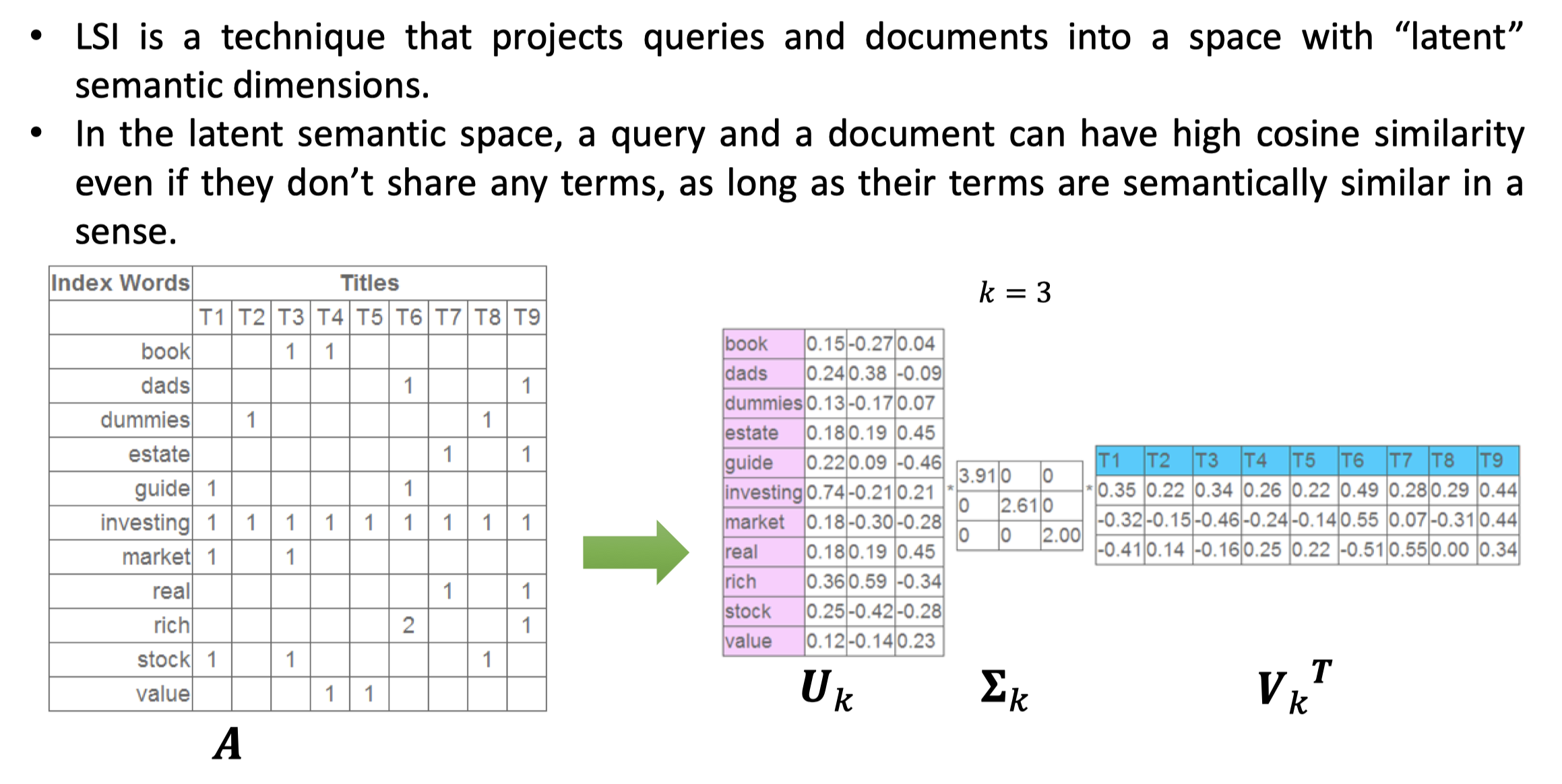更多 SVD 的应用：

展开全文正定矩阵 矩阵特征值分解 矩阵SVD分解 矩阵正交对角化
• 一、转置矩阵 假设矩阵 A 如下表示： ...若矩阵 B 与其转置矩阵相等，则称矩阵 B 为对称矩阵，如： 三、反对称矩阵 若矩阵 C 与其转置矩阵取负后相等，则称矩阵 C 为反对称矩阵，其对角线元素的值为0，如： ...

# 一、转置矩阵

### 假设矩阵 A 如下表示：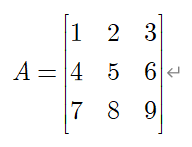### 则其转置矩阵表示为：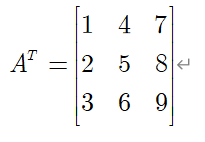# 二、对称矩阵

### 若矩阵 B 与其转置矩阵相等，则称矩阵 B 为对称矩阵，如：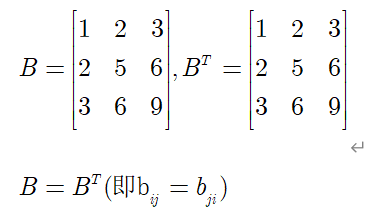# 三、反对称矩阵

### 若矩阵 C 与其转置矩阵取负后相等，则称矩阵 C 为反对称矩阵，其对角线元素的值为0，如：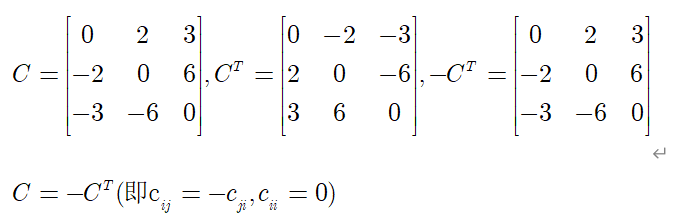# 四、向量的反对称矩阵

### 分别定义两个向量如下：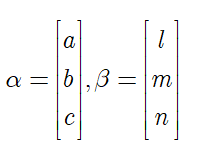### 对两个向量进行叉乘得到：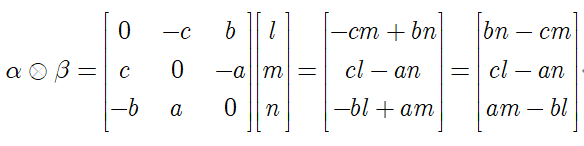### 则向量的反对称矩阵为：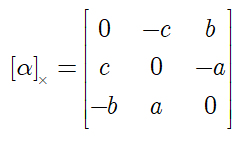展开全文• 线性代数之 实对称矩阵，正交对角化，二次型与正定矩阵前言实对称矩阵正交对角化二次型正定矩阵实对称矩阵的正定判断条件后记 前言 终于快到矩阵分解了。在矩阵分解前，最后一个内容是实对称矩阵，二次型和正定矩阵...

# 前言

终于快到矩阵分解了。在矩阵分解前，最后一个内容是实对称矩阵，二次型和正定矩阵。这三个概念与矩阵分解相关。

# 实对称矩阵

对于矩阵 A ∈ R n × n A\in R^{n\times n} ，如果 A T = A A^T=A ，则称 A A 为实对称矩阵。

实对称矩阵不同特征值的特征向量正交，n重特征值有n个线性无关的特征向量。因此实对称矩阵必然能够对角化。

实对称矩阵是 n × n n\times n 矩阵能够正交对角化的充分必要条件。

# 正交对角化

如果存在一个正交矩阵 Q Q ，使得方阵 A = Q Λ Q − 1 = Q Λ Q T A=Q\Lambda Q^-1=Q\Lambda Q^T 能够对角化，称为正交对角化。

能够正交对角化的矩阵都是对称矩阵。
证明：
A = Q Λ Q T A T = Q Λ T Q T = Q Λ Q T = A A=Q\Lambda Q^T \\ A^T=Q\Lambda^T Q^T=Q\Lambda Q^T=A

# 二次型

A A 是实对称矩阵，将一个变量满足 f ( x ) = x T A x f(x)=x^TAx 函数称为二次型。

对于 f ( x ) = x T A x f(x)=x^TAx ，替换变量 x = P y , f ( x ) = f ( P y ) = y T P T A P y x=Py,f(x)=f(Py)=y^TP^TAPy ，而 A A 是实对称矩阵，因此存在正交矩阵 Q , f ( Q y ) = y T Λ y Q,f(Qy)=y^T\Lambda y ，使得二次型化为标准型。

# 正定矩阵

广义的正定矩阵：对于矩阵 A ∈ R n × n A\in R^{n\times n} ，函数 f ( x ) = x T A x > 0 f(x)=x^TAx>0 对任意非零向量 x ∈ R n x\in R^n 都成立，则称 A A 为正定矩阵。如果 f ( x ) = x T A x ≥ 0 f(x)=x^TAx\ge0 ，则称 A A 为半正定矩阵。

狭义的正定矩阵：对于对称矩阵 A ∈ R n × n A\in R^{n\times n} ，函数 f ( x ) = x T A x > 0 f(x)=x^TAx>0 对任意非零向量 x ∈ R n x\in R^n 都成立，则称 A A 为正定矩阵。如果 f ( x ) = x T A x ≥ 0 f(x)=x^TAx\ge0 ，则称 A A 为半正定矩阵。也把这种正定矩阵称为对称正定矩阵。

## 实对称矩阵的正定判断条件

如果实对称矩阵的特征值都大于0，则是对称正定矩阵；如果特征值都非负，则是对称半正定矩阵。

证明：
f ( x ) = x T A x Q Q T = E x = Q y f ( x ) = f ( Q y ) = y T Q T A Q y = y T Λ y = ∑ i = 1 n λ i y i 2 i f λ i > 0 , f ( x ) > 0 i f λ i ≥ 0 , f ( x ) ≥ 0 f(x)=x^TAx \\ QQ^T=E \\ x=Qy\\ f(x)=f(Qy)=y^TQ^TAQy=y^T\Lambda y=\sum_{i=1}^n \lambda_i y_i^2 \\ if \quad \lambda_i>0,f(x)>0 \\ if \quad \lambda_i\ge0,f(x)\ge 0\\

## 一个常见的半正定矩阵

对于任意矩阵 A ∈ R m × n A\in R^{m\times n} 矩阵 A T A A^TA 是半正定矩阵

证明：
x T A T A x = ( A x ) T A x = ( A x ) ⋅ ( A x ) = ∣ ∣ A x ∣ ∣ 2 2 ≥ 0 x^TA^TAx=(Ax)^TAx=(Ax)\cdot(Ax)=||Ax||^2_2\ge0

如果 A ∈ R m × n A\in R^{m\times n} 列满秩，则 A T A A^TA 是正定矩阵

证明：
A x = 0 → A T A x = 0 A T A x = 0 → x T A T A x = 0 → ∣ ∣ A x ∣ ∣ 2 2 = 0 → A x = 0 ∴ N U L ( A ) = N U L ( A T A ) ∵ d i m N U L ( A ) + r a n k ( A ) = n ∴ r a n k ( A T A ) = r a n k ( A ) r a n k ( A ) = n , d i m N U L ( A ) = 0 ∴ ∀ x ≠ 0 , A x ≠ 0 ∴ ∣ ∣ A x ∣ ∣ 2 2 > 0 i . e . x T A T A x > 0 Ax=0 \to A^TAx=0 \\ A^TAx=0 \to x^TA^TAx=0 \to ||Ax||^2_2=0\to Ax=0 \\ \therefore NUL(A)=NUL(A^TA) \\ \because dimNUL(A)+rank(A)=n \\ \therefore rank(A^TA)=rank(A) \\ \quad \\ rank(A)=n,dimNUL(A)=0 \\ \therefore \forall x\ne 0, A x\ne0 \\ \therefore ||Ax||_2^2>0 \\ \quad \\ i.e. \quad x^TA^TAx>0

# 后记

线性代数的矩阵性质部分大概就记录完了。下一篇就进入到了矩阵计算的内容——矩阵分解。

展开全文机器学习
• 线性代数课程的一个重要特点(也是难点)是概念众多，而且各概念间有着千丝万缕的联系，对于初学者不易理解的问题我们会不惜笔墨加以解释。在内容上，以国内的经典教材“同济版线性代数”为蓝本，并适当选取了一些补充...
• 常见的一些特殊矩阵有：对称矩阵，上（下）三角矩阵和对角矩阵。特殊矩阵的压缩存储方法：找出特殊矩阵中值相等的元素的分布规律，把那些呈现规律性分布的，值相同的多个矩阵元素压缩存储到一个存储空间中： 特殊...数据结构
• 整理一下矩阵论学习中的相关概念。从正交矩阵开始正交矩阵 定义1 称n阶方阵A是正交矩阵，若 正交矩阵有几个重要性质： A的逆等于A的转置，即 A的行列式为±1，即 A的行（列）向量组为n维单位正交向量组上述3个性质...
• 本章是特征值与特征向量知识的延续，根据谱定理可知实对称矩阵可以正交对角化，对角阵为其特征值，正交矩阵为其两两正交的单位特征向量。除此之外，还介绍了二次型，标准形，规范形的知识。二次型的化简问题是本章的...
• 在许多实际问题中，所产生的矩阵往往都是对称矩阵，比如我们耳熟能详的实对称矩阵也是重要的研究对象。以下就从实对称矩阵的角度出发，利用特征值的极小极大原理，从普通特征值问题Ax=λxAx=\lambda xAx=λx衍生到...直积
• ## 什么是对称矩阵？

千次阅读 2021-07-01 22:39:31
对称矩阵（Symmetric Matrices）是指以主对角线为对称轴，各元素对应相等的矩阵。在线性代数中，对称矩阵是一个方形矩阵，其转置矩阵和自身相等。1855年，埃米特(C.Hermite,1822-1901年)证明了别的数学家发现的一些...
• 但是还原是不唯一的：二次型和一个实对称矩阵是可以对应的 简单的例子如下： 实对称矩阵 任何一个二次型，都可以写成 一横 一方 一竖 的形式： 就是 未知数 实对称矩阵 未知数： 现在在假设X=CY，其中C可逆 引入...
• 特征值和特征向量可能是线性代数中最重要的概念之一。从机器学习、量子计算、物理到许多数学和工程的问题，都可以通过找到一个矩阵的特征值和特征向量来解决。根据定义(标量λ、向量v是特征值、特征向量A)：视觉上，...
• ## 实对称矩阵性质的数学证明

万次阅读 多人点赞 2018-05-13 18:36:57
在进行实对称矩阵性质的数学证明之前，先证明一些会用到的有用理论。首先先引入复数的共轭概念： 假设z是复数(complex number)，z = a + bi，则z的共轭（conjugate of z）则写作z⎯⎯=a&amp;nbsp;−biz¯=a&...
• 符号对称矩阵的谱特征，刘玉霞，周继东，矩阵的谱特征是矩阵的一个重要性质，日益成为基础数学矩阵研究领域的一个很重要的研究方向。本文首先引入了一类特殊矩阵的概念
• 在次对称矩阵定义的基础上给出了K-(反)次对称矩阵概念,利用次对称矩阵的研究方法及特殊矩阵K的性质,推出了K-(反)次对称矩阵的若干性质,研究了几个与K-(反)次对称矩阵相关的问题,并讨论了K-次对称矩阵和K-次正交...
• 对称矩阵的特征值和特征向量 这一节，我们首先研究一类重要的矩阵，实对称矩阵，的特征值和特征向量。 性质 我们的主要结论是 实对称矩阵的特征值全部是实数。 实对称矩阵可以取到 nnn 个正交的特征实向量。 原因 ...向量空间
• 看文献的时候，经常见到各种各样矩阵，本篇总结了常见的对称矩阵、Hermite矩阵、正交矩阵、酉矩阵、奇异矩阵、正规矩阵、幂等矩阵七种矩阵的定义，作为概念备忘录吧，忘了可以随时查一下。 1、对称矩阵（文献【1】...
• 给出了次正交矩阵概念,研究了它的性质以及次正交矩阵与次对称阵、反次对称阵间的联系.
• 实际上是特征值的几何应用，概念仍需加强理解二次型:实际上是特征值的几何应用1、二次型化标准形:特征值、特征向量、相似对角化2、二次型的正定性3、合同:坐标变换正交变换化二次型为标准形，标准为求二次型矩阵 ...
• 特殊矩阵——对称矩阵(Symmetric Matrix) 注：压缩存储的矩阵可以分为...1. 对称矩阵概念 元素以主对角线为对称轴对应相等的矩阵。 2. 对称矩阵的特性 对角矩阵都是对称矩阵对称矩阵必须是方形矩阵。 设一个n数据结构 二维数组
• 对称矩阵、Hermite矩阵、正交矩阵、酉矩阵、奇异矩阵、正规矩阵、幂等矩阵 转载自：https://blog.csdn.net/jbb0523/article/details/50596604         看文献的时候，经常见到各种各样矩阵，本...数学
• 本文给出对称循环矩阵的概念，讨论了复对称矩阵的一般性质，并给出复对称循环阵的特征值的具体形状，还给出对称循环阵的求逆方法和分解定理．
• 给出了广义次对称(反次对称)矩阵和广义次正交矩阵概念,讨论了它们的性质及它们之间的关系。
• 本文讨论了一个在对称矩阵计算中有用的概念：户性质。给出了对称矩阵具有声性质的一个充分必要条件,以及在对称矩阵β具有产性质时,其常数声的确定。
• 引进了广义(R，S)-对称矩阵概念，给出了广义(R，S)-对称矩阵A的精确表达式，据此将方程AX=y化成若干低阶方程来求解.给出了广义(R，S)-对称矩阵A的几类广义逆的表达式.
• 本章是特征值与特征向量知识的延续，根据谱定理可知实对称矩阵可以正交对角化，对角阵为其特征值，正交矩阵为其两两正交的单位特征向量。除此之外，还介绍了二次型，标准形，规范形的知识。二次型的化简问题是本章的...
• 5.3实对称矩阵的对角化 正交矩阵 概念 A是一个n阶方阵 有A转置A == E，那么这个矩阵就是正交矩阵 性质 若A是正交矩阵，|A| == 1或 -1 若A是正交矩阵，A的逆 == A的转置 且A逆和A转置均为正交 A,B正交，AB也正交 若A...机器学习
•深度学习...